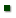Skip to main content
ARS Home » Research » Publications at this Location » Publication #95135

Title: SOLUTIONS FOR THREE REGRESSION PROBLEMS COMMONLY FOUND IN METEOROLOGICAL DATA ANALYSIS

AuthorMeek, DavidPrueger, JohnSauer, Thomas - Tom

 Submitted to: American Meteorological Society Publication Type: Proceedings Publication Acceptance Date: 11/6/1998 Publication Date: N/A Citation: N/A Interpretive Summary: When researchers analyze environmental data they often employ a tool called regression analysis. Unfortunately the basic assumptions of this tool are not commonly understood or verified. Through the presentation of three comparative examples, this work demonstrates how to identify and correctly analyze three common problems. The given examples all show considerable improvements in analysis results. Also, in addition, the correct analyses better meet the underlying statistical assumptions. Thus if the assumptions of ordinary regression are not sound then the relevant alternative methodology should be used instead. Researcher's decisions about results may change. Technical Abstract: The fundamental assumptions of regression analysis are ignored by all too many researchers in agricultural meteorology. Abuses are common in the literature! Through the presentation of three examples, this work demonstrates how to identify and correctly analyze three commonly found problems. The first example came from comparing simultaneous radiometer recordings from different radiometers. The problem was that the data were serially correlated. One solution to this type of problem is to use an autoregression. The second example came from an assessment of simultaneous evapotranspiration measurements. The problem was that the independent data were subject to random errors. The solution is to use measurement error methodology. The third example came from long-term precipitable water records. The problem was that the variability of the dependent variable was not constant over its range. The solution is to use a weighted regression analysis. These three examples represent common problems with solutions that demonstrate considerable improvements in analysis results. Generally interpolation and prediction are improved; moreover the underlying statistical assumptions are more tenable. Therefore when the assumptions of ordinary regression are not sound, the relevant alternative methodology should be used instead.